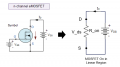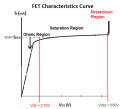# MOSFET very basic question

#### vcolo

Joined Feb 8, 2020
4
Hi all, question from somebody just starting to try to understand power electronics.

The basic specs of a power MOSFET are: Vdss(breakdown voltage) = 650V, Rds(ON)MAX= 99mOhms at 10V and IdMAX= 30A.

If the MOSFET was operating at maximum Vgs (30V by the specsheet), its ON resistance would be 99mOhms (probably lower but let's assume the spec sheet number for simplicity). If also I was pushing 30A through the MOSFET and assuming I am at the end of the linear region, would Vds be = Id x Rds(ON) = 30A x 0.099 = 2.97V?

If so, it seems strange to me that Vdss = 650V. Why that margin of 647V until breakdown?

Thanks!

#### crutschow

Joined Mar 14, 2008
30,459
it seems strange to me that Vdss = 650V. Why that margin of 647V until breakdown?
Vdss is the maximum off-voltage that can be applied from the source to drain of the MOSFET.
It determines the maximum supply voltage and/or transient voltage that can be applied to the device.
It has no direct relation to the on-resistance or on-voltage.
Why do you think there should be?

#### vcolo

Joined Feb 8, 2020
4
- When a MOSFET is in ON state and in the linear region, it behaves as a resistance with a value the on-resistance. Therefore, if there is a current Id going through the device, the voltage between source and drain is Vds = Id x R_on-When I operate the MOSFET at its maximum Id spec and minimum R_on, I assume I am at the end of the linear region. Based on the previous point Vds = Id_max x R_on = 30A x 0.099Ohms =2.97V
-If then I increase Vds, Id will remain aprox. constant (saturation region). I can keep increasing Vds until I reach the breakdown value Vdss = 650V, but I won't get more performance out of the device.Feel free to tell me I got any of the concepts above wrong please. Based on the stuff explained, I have a hard time reasoning the 650V. If you have a transistor that won't get any extra performance or benefit being operated beyond 2.76V, ok it is good to know it will break after 650V but it's so far away that I feel the risk of that happening is very low.

However, since Vdss is the first spec every manufacturer puts in their sheets, I must be wrong.